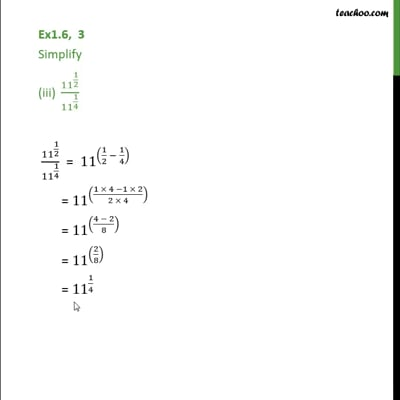Ex 1.6

Chapter 1 Class 9 Number Systems
Serial order wiseThis video is only available for Teachoo black users

Maths Crash Course - Live lectures + all videos + Real time Doubt solving!

### Transcript

Ex1.6, 3 Simplify (iii) 11 ^(1/2)/ 11 ^(1/4) 11 ^(1/2)/ 11 ^(1/4) = 11 ^((1/2 1/4) ) = 11 ^(((1 4 1 2)/(2 4)) ) = 11 ^(((4 2)/8) ) = 11 ^((2/8) ) = 11 ^(1/4 )